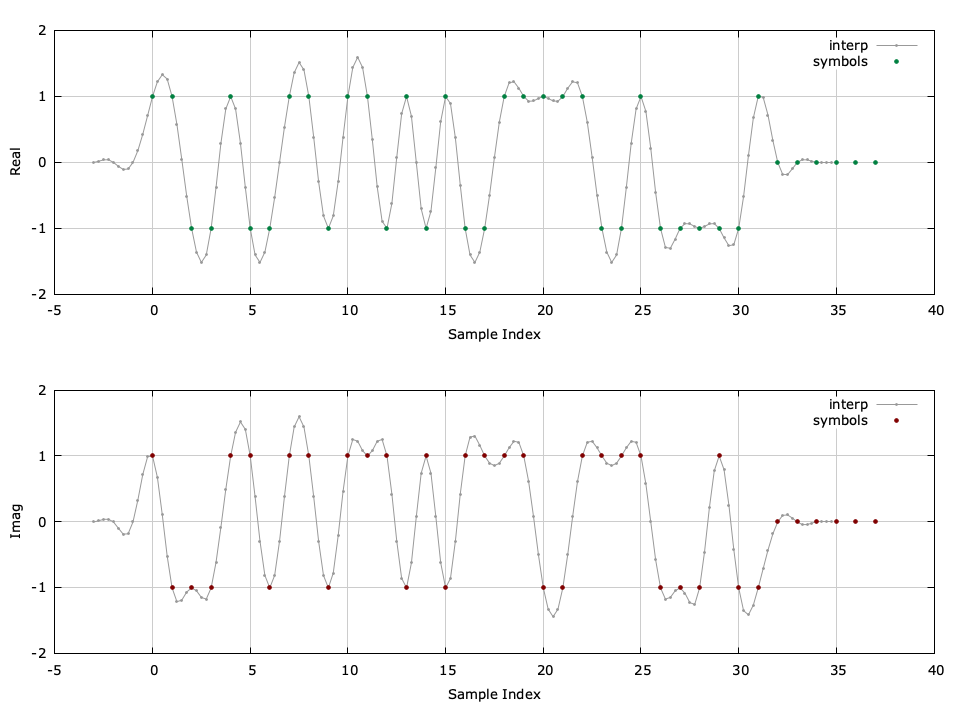# Finite Impulse Reseponse Interpolator (firinterp)

API Keywords: firinterp interpolation resample

The interp object implements a basic interpolator with an integer output-to-input resampling ratio. An example of the interp interface is listed below.

#include <liquid/liquid.h>

int main() {
unsigned int M=4;       // interpolation factor
unsigned int h_len;     // interpolation filter length

// design filter and create interpolator
float h[h_len];         // filter coefficients
firinterp_crcf q = firinterp_crcf_create(M,h,h_len);

// generate input signal and interpolate
float complex x;        // input sample
float complex y[M];     // output samples

// run interpolator (repeat as necessary)
{
firinterp_crcf_execute(q, x, y);
}

// destroy the interpolator object
firinterp_crcf_destroy(q);
}


Listed below is the full interface to the interp family of objects. While each method is listed for firinterp_crcf , the same functionality applies to interp_rrrf and interp_cccf .

• firinterp_crcf_create(M,*h,N) creates an interp object with an interpolation factor $$M$$ using $$N$$ filter coefficients $$\vec{h}$$ .
• firinterp_crcf_create_prototype(M,m,As) create an interp object using a filter prototype designed using the firdes_kaiser_window() method (see [ref:section-filter-firdes] ) with a normalized cut-off frequency $$1/2M$$ , a filter length of $$2Mm$$ coefficients, and a stop-band attenuation of $$A_s$$ dB.
• firinterp_crcf_create_rnyquist(type,k,m,beta,dt) creates an interp object from a square-root Nyquist filter prototype with$$k$$ samples per symbol (interpolation factor),$$m$$ symbols of delay,$$\beta$$ excess bandwidth, and a fractional sampling interval $$\Delta t$$ .[ref:section-filter-firdes-rnyquist] provides a detailed description of the available square-root Nyquist filter prototypes available in liquid.
• firinterp_crcf_destroy(q) destroys the interpolator, freeing all internally-allocated memory.
• firinterp_crcf_print(q) prints the internal properties of the interpolator to the standard output.
• firinterp_crcf_clear(q) clears the internal interpolator buffers.
• firinterp_crcf_execute(q,x,*y) executes the interpolator for an input $$x$$ , storing the result in the output array $$y$$ (which has a length of $$M$$ samples).Figure [fig-filter-firinterp_crcf]. firinterp_crcf (interpolator) example with $$M=4$$ , compensating for filter delay.

A graphical example of the interpolator can be seen in[ref:fig-filter-firinterp_crcf] . A detailed example program is given in examples/firinterp_crcf_example.c , located under the main liquid project directory.